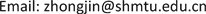1. 引言

min x max i f i ( x ) .

p i ( i = 1 , 2 , ⋯ , m ) ，平面上任一点x到各点的距离则分别为 ‖ x − p i ‖ ( i = 1 , 2 , ⋯ , m ) 。若以 f ( x ) 表示x到m个点 p i 的最大距离，即 f ( x ) = max 1 ≤ i ≤ m ‖ x − p i ‖ ，则以x为圆心，以 f ( x ) 为半径的圆盘能覆盖这m个点。于

min x ∈ R 2 f ( x ) = min x ∈ R 2 max 1 ≤ i ≤ m ‖ x − p i ‖ ,

min x ∈ D φ [ f ( x ) ] = min x ∈ D max 1 ≤ i ≤ p f i ( x ) .

2. 模型建立2.1. 多目标规划模型

f ( x ) = ( f 1 ( x ) , f 2 ( x ) , f 3 ( x ) ) ，其中第一个目标是挖坑

f 1 ( x ) = 20 x 1 + 10 x 4 ,

f 2 ( x ) = 30 x 2 + 20 x 5 ,

f 3 ( x ) = 25 x 3 + 15 x 6 ,

x 1 + x 2 + x 3 ≤ 30 ,

x 4 + x 5 + x 6 ≤ 20 ,

max 20 x 1 + 10 x 4 max 30 x 2 + 20 x 5 max 25 x 3 + 15 x 6

s .t .   25 x 3 + 15 x 6 ≤ 20 x 1 + 10 x 4 ,     25 x 3 + 15 x 6 ≤ 30 x 2 + 20 x 5 ,     x 1 + x 2 + x 3 ≤ 30 ,     x 4 + x 5 + x 6 ≤ 20 ,     其 中 x 1 , x 2 , x 3 , x 4 , x 5 , x 6 都 为 整 数

2.2. 极小极大问题模型

min max 1 ≤ i ≤ 3 f i ( x ) s .t .   x 1 + x 2 + x 3 ≤ 30 ,     x 4 + x 5 + x 6 ≤ 20 ,     其 中 x 1 , x 2 , x 3 , x 4 , x 5 , x 6 都 为 整 数

3. 模型的求解

max   s s .t .     20 x 1 + 10 x 4 ≥ s ,     30 x 2 + 20 x 5 ≥ s ,     25 x 3 + 15 x 6 ≥ s ,

x 1 + x 2 + x 3 ≤ 30 , x 4 + x 5 + x 6 ≤ 20 , 其 中 x 1 , x 2 , x 3 , x 4 , x 5 , x 6 都 为 整 数

4. 结果的验证和分析4.1. 结果的验证

min x max i f i ( x ) = − max x min i ( − f i ( x ) ) ,

− max min 1 ≤ i ≤ 3 ( − f i ( x ) ) s .t .   x 1 + x 2 + x 3 ≤ 30     x 4 + x 5 + x 6 ≤ 20     x 1 , x 2 , x 3 , x 4 , x 5 , x 6 都 为 整 数

− min   − s s .t .     − 20 x 1 − 10 x 4 ≤ − s ,     − 30 x 2 − 20 x 5 ≤ − s ,     − 25 x 3 − 15 x 6 ≤ − s ,

x 1 + x 2 + x 3 ≤ 30 , x 4 + x 5 + x 6 ≤ 20 , 其 中 x 1 , x 2 , x 3 , x 4 , x 5 , x 6 都 为 整 数

4.2. 结果的分析

max s = − min   − s ,

20 x 1 + 10 x 4 ≥ s , 30 x 2 + 20 x 5 ≥ s , 25 x 3 + 15 x 6 ≥ s ,

− 20 x 1 − 10 x 4 ≤ − s , − 30 x 2 − 20 x 5 ≤ − s , − 25 x 3 − 15 x 6 ≤ − s ,

5. 总结